• 题目：定义Fibonacci数列如下：  / 0 n=0 f(n)= 1 n=1  \ f(n-1)+f(n-2) n=2 输入n，用最快的方法求该数列的第n项。...分析：在很多C语言教科书中讲到递归函数的时候，都会用Fibonacci作为例子。因
题目：定义Fibonacci数列如下：
/  0                      n=0
f(n)=      1                      n=1        \  f(n-1)+f(n-2)          n=2
输入n，用最快的方法求该数列的第n项。
分析：在很多C语言教科书中讲到递归函数的时候，都会用Fibonacci作为例子。因此很多程序员对这道题的递归解法非常熟悉，看到题目就能写出如下的递归求解的代码。
///// Calculate the nth item of Fibonacci Series recursively///long long Fibonacci_Solution1(unsigned int n){      int result
= {0, 1};      if(n
< 2)            return result[n];      return Fibonacci_Solution1(n
- 1) + Fibonacci_Solution1(n - 2);}
但是，教科书上反复用这个题目来讲解递归函数，并不能说明递归解法最适合这道题目。我们以求解f(10)作为例子来分析递归求解的过程。要求得f(10)，需要求得f(9)和f(8)。同样，要求得f(9)，要先求得f(8)和f(7)……我们用树形结构来表示这种依赖关系
f(10)               /        \            f(9)         f(8)          /     \       /    \       f(8)     f(7)  f(7)   f(6)      /   \     /   \    f(7)  f(6)  f(6) f(5)
我们不难发现在这棵树中有很多结点会重复的，而且重复的结点数会随着n的增大而急剧增加。这意味这计算量会随着n的增大而急剧增大。事实上，用递归方法计算的时间复杂度是以n的指数的方式递增的。大家可以求Fibonacci的第100项试试，感受一下这样递归会慢到什么程度。在我的机器上，连续运行了一个多小时也没有出来结果。
其实改进的方法并不复杂。上述方法之所以慢是因为重复的计算太多，只要避免重复计算就行了。比如我们可以把已经得到的数列中间项保存起来，如果下次需要计算的时候我们先查找一下，如果前面已经计算过了就不用再次计算了。
更简单的办法是从下往上计算，首先根据f(0)和f(1)算出f(2)，在根据f(1)和f(2)算出f(3)……依此类推就可以算出第n项了。很容易理解，这种思路的时间复杂度是O(n)。
///// Calculate the nth item of Fibonacci Series iteratively///long long Fibonacci_Solution2(unsigned n){      int result
= {0, 1};      if(n
< 2)            return result[n];      long long  fibNMinusOne
= 1;      long long  fibNMinusTwo
= 0;      long long  fibN
= 0;      for(unsigned int i
= 2; i <= n; ++ i)      {            fibN
= fibNMinusOne + fibNMinusTwo;            fibNMinusTwo
= fibNMinusOne;            fibNMinusOne
= fibN;      }
return fibN;}
这还不是最快的方法。下面介绍一种时间复杂度是O(logn)的方法。在介绍这种方法之前，先介绍一个数学公式：
{f(n), f(n-1), f(n-1), f(n-2)} ={1, 1, 1,0}n-1
(注：{f(n+1), f(n), f(n), f(n-1)}表示一个矩阵。在矩阵中第一行第一列是f(n+1)，第一行第二列是f(n)，第二行第一列是f(n)，第二行第二列是f(n-1)。)
有了这个公式，要求得f(n)，我们只需要求得矩阵{1, 1, 1,0}的n-1次方，因为矩阵{1,
1, 1,0}的n-1次方的结果的第一行第一列就是f(n)。这个数学公式用数学归纳法不难证明。感兴趣的朋友不妨自己证明一下。
现在的问题转换为求矩阵{1, 1, 1, 0}的乘方。如果简单第从0开始循环，n次方将需要n次运算，并不比前面的方法要快。但我们可以考虑乘方的如下性质：
/  an/2*an/2                      n为偶数时an=        \  a(n-1)/2*a(n-1)/2            n为奇数时
要求得n次方，我们先求得n/2次方，再把n/2的结果平方一下。如果把求n次方的问题看成一个大问题，把求n/2看成一个较小的问题。这种把大问题分解成一个或多个小问题的思路我们称之为分治法。这样求n次方就只需要logn次运算了。
实现这种方式时，首先需要定义一个2×2的矩阵，并且定义好矩阵的乘法以及乘方运算。当这些运算定义好了之后，剩下的事情就变得非常简单。完整的实现代码如下所示。
#include ///// A 2 by 2 matrix///struct Matrix2By2{      Matrix2By2      (            long long m00
= 0,             long long m01
= 0,             long long m10
= 0,             long long m11
= 0      )      :m_00(m00), m_01(m01), m_10(m10),
m_11(m11)       {      }      long long m_00;      long long m_01;      long long m_10;      long long m_11;};///// Multiply two matrices// Input: matrix1 - the first matrix//        matrix2
- the second matrix//Output: the production of two matrices///Matrix2By2 MatrixMultiply(      const Matrix2By2&
matrix1,       const Matrix2By2&
matrix2){      return Matrix2By2(            matrix1.m_00
* matrix2.m_00 + matrix1.m_01 * matrix2.m_10,            matrix1.m_00
* matrix2.m_01 + matrix1.m_01 * matrix2.m_11,            matrix1.m_10
* matrix2.m_00 + matrix1.m_11 * matrix2.m_10,            matrix1.m_10
* matrix2.m_01 + matrix1.m_11 * matrix2.m_11);}///// The nth power of matrix // 1  1// 1  0///Matrix2By2 MatrixPower(unsigned int n){      assert(n > 0);      Matrix2By2 matrix;      if(n
== 1)      {            matrix
= Matrix2By2(1, 1, 1, 0);      }      else if(n
% 2 == 0)      {            matrix
= MatrixPower(n / 2);            matrix
= MatrixMultiply(matrix, matrix);      }      else if(n
% 2 == 1)      {            matrix
= MatrixPower((n - 1) / 2);            matrix
= MatrixMultiply(matrix, matrix);            matrix
= MatrixMultiply(matrix, Matrix2By2(1, 1, 1, 0));      }      return matrix;}///// Calculate the nth item of Fibonacci Series using devide and conquer///long long Fibonacci_Solution3(unsigned int n){      int result
= {0, 1};      if(n
< 2)            return result[n];      Matrix2By2 PowerNMinus2 = MatrixPower(n
- 1);      return PowerNMinus2.m_00;}

转载自：http://blog.sina.com.cn/s/blog_6ab0b9a80101axdn.html

展开全文• 斐波那契数列 递归算法 循环算法 优化递归算法 以及它们的时间复杂度，空间复杂度分析
斐波那契数列

【含义】：

斐波那契数列，又称黄金分割数列，指的是这样一个数列：0、1、1、2、3、5、8、13、21、34、……在数学上，斐波纳契数列以如下被以递归的方法定义：F（0）=0，F（1）=1，F（n）=F(n-1)+F(n-2)（n≥2，n∈N*）在现代物理、准晶体结构、化学等领域，斐波纳契数列都有直接的应用，为此，美国数学会从1963起出版了以《斐波纳契数列季刊》为名的一份数学杂志，用于专门刊载这方面的研究成果。

用C求取第n个斐波那契数

【时间复杂度】：递归总次数*每次递归的次数

【空间复杂度】：递归的深度*每次递归空间的大小

【递归深度】：树的高度（递归的过程是一个”二叉树”）

【一般算法O(n)计算方法】：
- 用常数1取代运行时间中的所有加法常数
- 在修改后的运行次数函数中，只保留最高阶项
- 如果最高阶项系数存在且不是1，则去除与这个项相乘的常数。

【普通递归实现】：

#include<stdio.h>
#include<Windows.h>

long long fib(long long n){
if(n < 3)
return 1;
return fib(n-1)+fib(n-2);
}

int main(){
long long n = 0;
scanf("%llu",&n);
printf("%llu\n",fib(n));
system("pause");
return 0;
}

【时间复杂度】：O(2^n)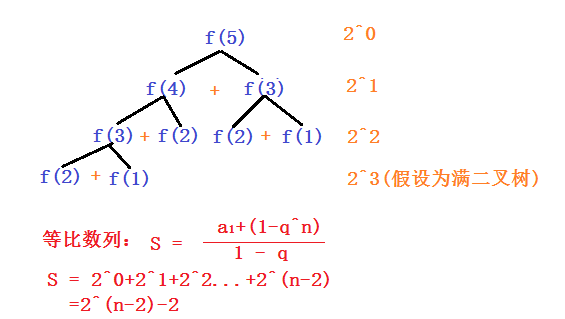【空间复杂度】：O(n)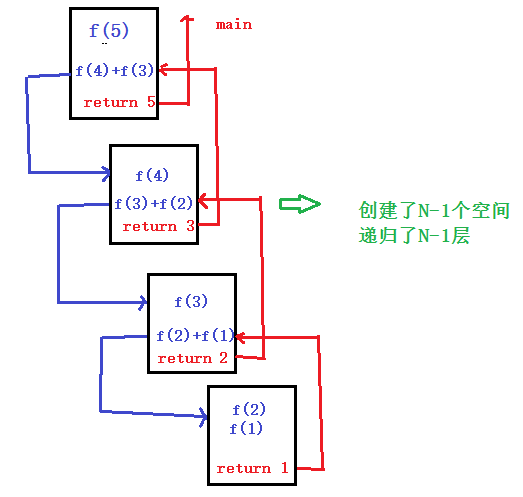【缺陷】：重复计算次数太多，效率低下。

【循环】：

#include<stdio.h>
#include<Windows.h>

long long fib(long long n){
int i = 0;
long long first = 1,second = 1;
long long ret = 0;
for(i=3;i<=n;++i){
ret = first + second;
first = second;
second = ret;
}
return second;
}

int main(){
long long n = 0;
scanf("%llu",&n);
printf("%llu\n",fib(n));
system("pause");
return 0;
}

【时间复杂度】：O(n)
【空间复杂度】：O(1)

【升级版递归】：

#include<stdio.h>
#include<Windows.h>

long long fib(long long first,long long second,long long n){
if(n < 3)
return 1;
if(n == 3)
return first + second;
return fib(second,first+second,n-1);
}

int main(){
long long n = 0;
scanf("%llu",&n);
printf("%llu\n",fib(1,1,n));
system("pause");
return 0;
}

【分析】：

本算法采用了尾递归的算法；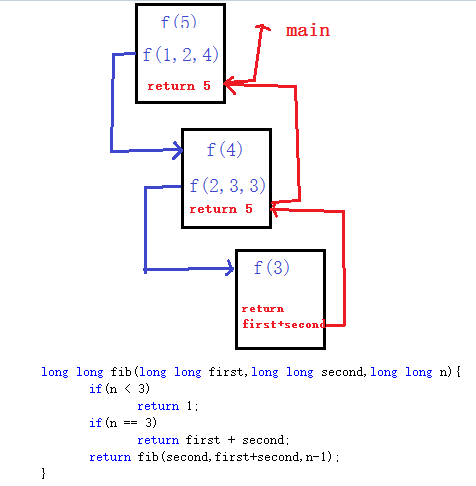【时间复杂度】：O(n)

【空间复杂度】：O(n) （在VS debug环境下，其他环境有可能会进行编译器优化）

【注意】：尾递归有时候在特定环境下会产生编译器优化，即不会再为尾递归函数调用下一级函数时开辟新栈，而是直接在旧函数的内存块上进行修改），这时它的空间复杂度为O(1)。
展开全文递归 优化
• 一：递归实现  使用公式f[n]=f[n-1]+f[n-2]，依次递归计算... 当然队列比数组更适合实现斐波那契数列时间复杂度和空间复杂度和vector一样，但队列太适合这里了，  f(n)=f(n-1)+f(n-2)，f(n)只和f(n-1)和f(n-2)有关
• 斐波那契数列指的是这样一个数列：0 、1、1、2、3、5、8、13、21，后面的每一个数是前面两个数的和。（由于数列增长快，int型就只能算到近50位，所以尽可能采用long long型）* 方法一：递归实现 /...
题目：

使用递归和非递归的方法分别实现求第n个斐波那契数，那么什么是斐波那契数。斐波那契数列指的是这样一个数列：0 、1、1、2、3、5、8、13、21，后面的每一个数是前面两个数的和。（由于数列增长快，int型就只能算到近50位，所以尽可能采用long long型）*方法一：递归实现
//时间复杂度O(N^2)
//空间复杂度o(N)
long long fib(size_t n)
{
return (n < 2) ? n : (fib(n - 1) + fib(n - 2));
}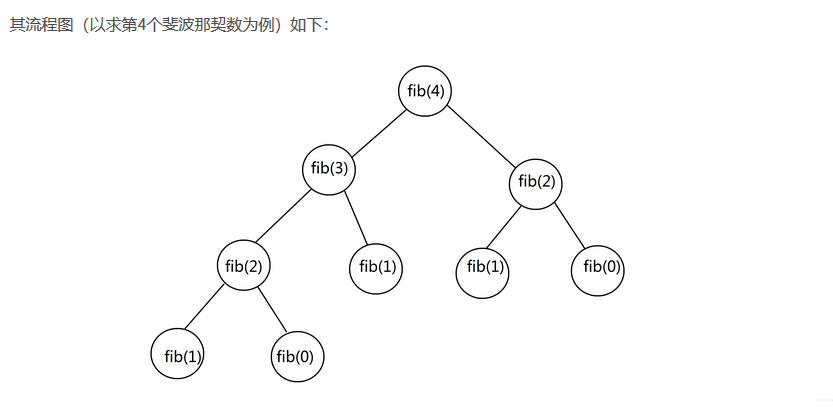缺点：
①只是使用于n比较小的时候，否则效率低，因为会做很多次重复操作
②而且该例递归属于多分支递归，容易造成栈溢出
方法二：非递归实现（迭代）
//时间复杂度O(N)
//空间复杂度O(1)
long long fib(size_t n)
{
long long n1 = 0,n2= 1,n3=n;
int i = 0;
for(i = 2;i<=n;i++)
{
n3 = n1+n2;
n1 = n2;
n2 = n3;
}
return n3;
}

方法三：尾递归实现
//时间复杂度O（N）
long long fib(long long first,long long second,size_t n)
{
if(n<2)
{
return n;
}
if(2 == n)
{
return first+second；
}
return fib(second,first+second,n-1);

}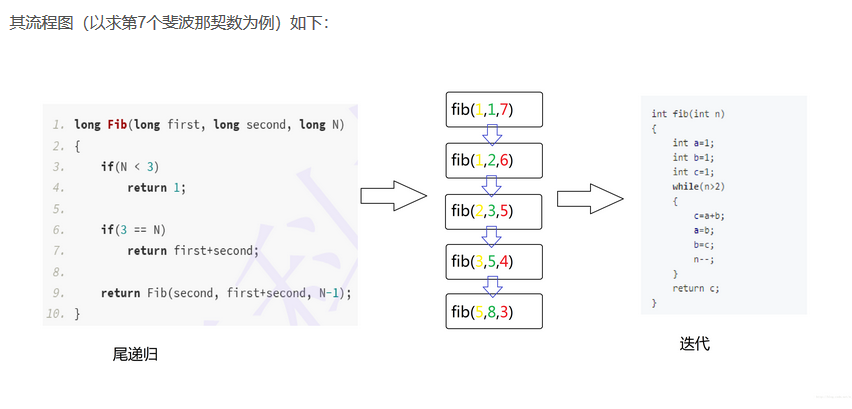优点：
①计算结果参与到下一次的计算，从而减少很多重复计算量
②原本朴素的递归产生的栈的层次像二叉树一样，以指数级增长，但是现在栈的层次却像是数组，变成线性增长了，简单来说，原本栈是先扩展开，然后边收拢边计算结果，现在却变成在调用自身的同时通过参数来计算。
尾递归可以转换成迭代算法
总结：
尾递归的本质是：将单次计算的结果缓存起来，传递给下次调用，相当于自动累积
方法四：矩阵乘法实现 (最优解)

常识：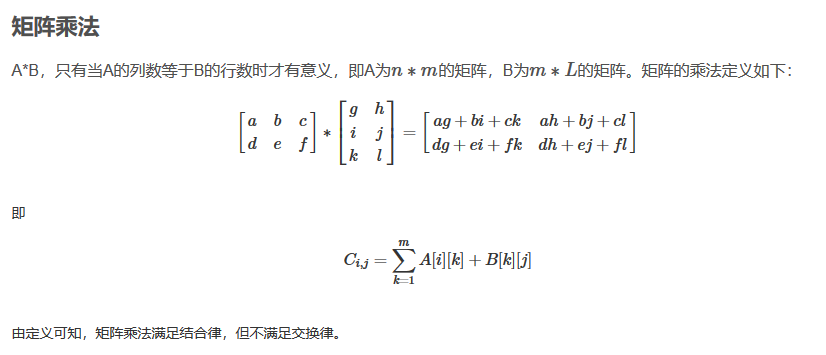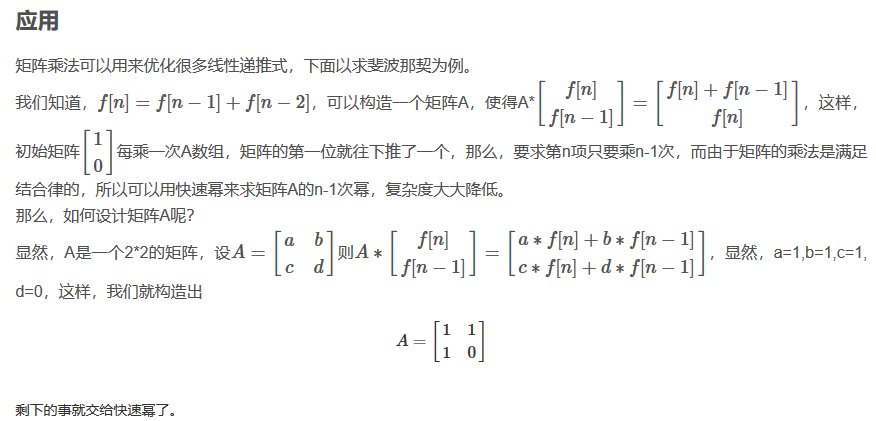快速幂：

#include <stdio.h>
//base 底数 ，exp 指数
int qpow(int base,int exp)
{
if (0==exp ) return 1;

int ret=1;

while(exp)
{
if(exp&1)//exp最右边一位 按位与&
{
ret=ret*base;
}
base=base*base;
exp>>=1;//右移一位
}
return ret;
}

int main()
{
printf("%d",qpow(3,5));
return 0;
}

注：此方法借鉴与 谷歌面试题精选
#include<iostream>
#include<string>
using namespace std;

//定义2×2矩阵；
struct Matrix2by2
{
//构造函数
Matrix2by2
(
long m_00,
long m_01,
long m_10,
long m_11
)
:m00(m_00),m01(m_01),m10(m_10),m11(m_11)
{
}

//数据成员
long m00;
long m01;
long m10;
long m11;
};

//定义2×2矩阵的乘法运算
Matrix2by2 MatrixMultiply(const Matrix2by2& matrix1,const Matrix2by2& matrix2)
{
Matrix2by2 matrix12(1,1,1,0);
matrix12.m00 = matrix1.m00 * matrix2.m00 + matrix1.m01 * matrix2.m10;
matrix12.m01 = matrix1.m00 * matrix2.m01 + matrix1.m01 * matrix2.m11;
matrix12.m10 = matrix1.m10 * matrix2.m00 + matrix1.m11 * matrix2.m10;
matrix12.m11 = matrix1.m10 * matrix2.m01 + matrix1.m11 * matrix2.m11;
return matrix12;

}

//定义2×2矩阵的幂运算
Matrix2by2 MatrixPower(unsigned int n)
{
Matrix2by2 matrix(1,1,1,0);
if(n == 1)
{
matrix = Matrix2by2(1,1,1,0);
}
else if(n % 2 == 0)
{
matrix = MatrixPower(n / 2);
matrix = MatrixMultiply(matrix, matrix);
}
else if(n % 2 == 1)
{
matrix = MatrixPower((n-1) / 2);
matrix = MatrixMultiply(matrix, matrix);
matrix = MatrixMultiply(matrix, Matrix2by2(1,1,1,0));
}
return matrix;
}
//计算Fibnacci的第n项
long Fibonacci(unsigned int n)
{
if(n == 0)
return 0;
if(n == 1)
return 1;

Matrix2by2 fibMatrix = MatrixPower(n-1);
return fibMatrix.m00;

}

int main()
{
cout<<"Enter A Number:"<<endl;
unsigned int number;
cin>>number;
cout<<Fibonacci(number)<<endl;
return 0;
}


结语：

努力的人生是完美的，因为不曾后悔过！！！


展开全文递归 矩阵乘法 尾递归
• 提起斐波那契数列，首先想到的大概都是递归，但是其时间复杂度并非最优，其时间复杂度为O(2^N)。具体分析可以参考：Fibonacci 方法二 循环 递归之所以效率低下，是因为需要重复的计算一些中间变量。而利用循环可以...
方法一 递归
提起斐波那契数列，首先想到的大概都是递归，但是其时间复杂度并非最优，其时间复杂度为O(2^N)。具体分析可以参考：Fibonacci
方法二 循环
递归之所以效率低下，是因为需要重复的计算一些中间变量。而利用循环可以通过存储中间变量来减小计算量，其时间复杂度为O(N)。

方法三 矩阵乘法
利用矩阵乘法加上分治的思想，可以将其时间复杂度降低到O(log2^n).具体的代码如下。关于其思路分析可以参考：Fibonacci

#include<stdio.h>

typedef struct MTX{
int m00;
int m01;
int m10;
int m11;
}mtx;
mtx mtx0;
mtx m_multiply(mtx a, mtx b)
{
mtx c;
c.m00=a.m00*b.m00+a.m01*b.m10;
c.m01=a.m00*b.m01+a.m01*b.m11;
c.m10=a.m10*b.m00+a.m11*b.m10;
c.m11=a.m10*b.m01+a.m11*b.m11;
return c;
}

mtx m_power(int k)
{
mtx m;

if(1==k)
{
m=mtx0;
return m;
}
else if(0==k%2)
{
m=m_multiply(m_power(k/2),m_power(k/2));
return m;
}
else
{
m=m_multiply(m_power((k-1)/2),m_power((k-1)/2));
m=m_multiply(m,mtx0);
return m;
}
}
int fibonacci(int n)
{
int m;
mtx mtx1;

int t={0, 1};
if(n<3)
return t[n];
else
mtx1=m_power(n-2);
return mtx1.m00;
}

int main(void)
{
mtx0.m00=1;
mtx0.m01=1;
mtx0.m10=1;
mtx0.m11=0;
int n=8;

int m=fibonacci(n);
printf("Fibonacci(%d)=%d",n,m);
return m;
}

展开全文Fibonacci 算法
• 问题描述：求解斐波那契数列，分别采用递归方式与非递归方式 方式一：递归方式 优点：代码简洁，易读 缺点：由于递归时上一个结果需要用到后面的计算，就会进行嵌套，且存在重复计算，时间复杂度为O(2^n),...算法
• 本篇是第9题_斐波那契数列C语言实现： 题目描述：大家都知道斐波那契数列，现在要求输入一个整数n，请你输出斐波那契数列的第n项。 用递归方法实现的代码量很小，但是时间复杂度是O（n^2）,本文采用循环实现，时间...剑指offer
• 实验内容： ①利用多种方法实现斐波那契数列分别可用循环，递归和分治3种方法，并由此估算三种算法的时间复杂度，比较三种算法的运行效率。 ②首先定义主函数分别定义3个时间变量来作为三种算法的运行时间，并且在...算法 函数 递归
• 什么是递推公式 递推公式就是形如斐波那契数列那样...学习C语言后我们都知道斐波那契数列的递归求法，很好理解： 1.递归求法 时间复杂度O(2n) int Fibonacci(int n){ return n>2?Fibonacci(n-1)+Fibonacci(n-2)...
• 题目一：写一个函数，输入n, 求斐波那契（Fibonacci）数列的第n项...分析：这种方法虽然直观但是时间效率很低， 因为重复的计算过多，计算量会随着n的增大急剧增大， 时间复杂度以 n 的指数方式递增 long long...
• 解析：一直以来很多C语言教科书在讲递归函数的时候总会拿斐波那契数列作为例子。但是这不意味这Fibonacci的最合适的解法就是用递归来实现。如果面试问道这题然后用递归解，那基本就GG了。因为递归调用过程中有很多...C++ 算法 剑指Offer 递归 面试题
•  构建Fibonacci序列，分别用递归、递推、数组构建Fibonacci序列的若干项，分别从时间、空间的复杂度角度对三种方法进行比较，并实际测试三种方法程序运行的时间C语言代码： #include #include #include int ...
• 解法一:刚进大一的时候,学习c语言,斐波那契数列是经常用于展示递归的经典例子.但是从时间复杂度角度来说的话,采用尾递归这并不是一个优秀的算法.public void Fibonacci(int n){ if(n){ return 0; } if(n==1){ ...
• 在大一的时候，初学c语言，我们就学过了关于斐波那契数列的计算，但是当时学的递归的方法计算起比较大的数值n，电脑会跑很久甚至都跑不动。 这次就用一个优化后的计算方法与递归方法作对比，通过一些例子学会计算...java 数据结构 算法
• 斐波那契数列（Fibonaccisequnce），又称黄金分割...第一种利用递归的方法计算，代码相当简单，但其重复计算率太高，导致其时间复杂度比指数还要爆炸式增长。不推荐此方法。但递归的思想却相当的重要还是要理解并掌...
• 1，斐波那契数列 ①递归 时间复杂度O(2^n)#include <stdio.h> int fib(int n){ if(n==1||n==2) return 1; return fib(n-1) + fib(n-2); } int main(){ int n; scanf("%d",&n); printf(...
• 大家都知道斐波那契数列，现在要求输入一个整数n，请你输出斐波那契数列的第n项。 大概所有同学C语言课程都敲过吧，那么这里我就不说那两个简单的方法，递归和迭代。 但是比较大的情况呢，甚至超过int范围，这就要...
• 剑指Offer 10-Ⅰ 斐波那契数列 10-Ⅱ青蛙跳台阶问题 方法：递归 和 迭代 递归超出时间 迭代注意取模值 C语言中规定%(取模)运算符的两个操作数必须同为整数类型 注意 1e9+7被系统认作浮点数，因此...算法 dfs leetcode
• 在分析一个程序算法时间复杂度时，可以使用统计程序或程序片段的计算时间有助于理解程序性质。许多语言或系统都提供了内部计时功能。有关c语言的如下： 有关函数在time.h头文件中，在程序头部写成：#include&lt...
• 4.8.1斐波那契数列实现 101 4.8.2递归定义 103 4.9栈的应用——四则运算表达式求值 104 4.9.1后缀（逆波兰）表示法定义 104 4.9.2后缀表达式计算结果 106 4.9.3中缀表达式转后缀表达式 108 4.10队列的定义 111...数据结构
• 2. 算法的时间复杂度取决于（C ）【中科院计算所 1998 二、1 （2分）】 A．问题的规模 B. 待处理数据的初态 C. A和 B 3.计算机算法指的是（C），它必须具备（B） 这三个特性。 (1) A．计算方法 B. 排序方法 C. 解决...
• ## C++程序员面试宝典

热门讨论 2013-04-01 13:36:19
许多开发者对C/C++语言及其底层原理掌握不牢固，在面试过程中经常漏洞百出，无法取得好成绩。而招聘单位为了得到高素质的员工往往采用各种形式的面试考察求职者，这让面试难度大大增加。求职者要想成功应聘，不仅...C++面试宝典# 斐波那契数列c语言时间复杂度c语言 订阅